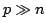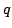Statistica Sinica 20 (2010), 595-611

ON MODEL SELECTION CONSISTENCY OF

THE ELASTIC NET WHENJinzhu Jia and Bin Yu

Peking University and University of California, Berkeley

Abstract: We study the model selection property of the Elastic Net. In the classical settings when(the number of predictors) and(the number of predictors with non-zero coefficients in the true linear model) are fixed, Yuan and Lin (2007) give a necessary and sufficient condition for the Elastic Net to consistently select the true model. They showed that it consistently selects the true model if and only if there exist suitable sequencesandthat satisfy EIC (which is defined later in the paper). Here we study the general case when, andall go to infinity. For general scalings of, and, when gaussian noise is assumed, sufficient conditions are given such that EIC guarantees the Elastic Net's model selection consistency. We show that to make these conditions hold,should grow at a rate faster than. We compare the variable selection performance of the Elastic Net with that of the Lasso. Through theoretical results and simulation studies, we provide insights into when the Elastic Net can consistently select the true model even when the Lasso cannot. We also point out through examples that when the Lasso cannot select the true model, it is very likely that the Elastic Net cannot select the true model either.

Key words and phrases: Elastic irrepresentable condition, Elastic Net, irrepresentable condition, Lasso, model selection consistency.